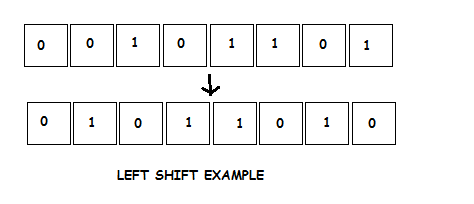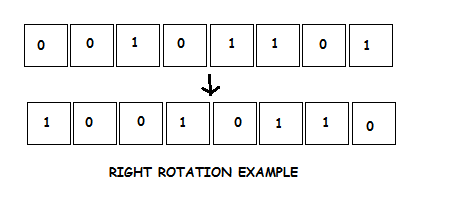×

Search anything:

# Left and Right Bit Rotation using Bitwise Operators

#### bitwise operation AlgorithmsGet this book -> Problems on Array: For Interviews and Competitive Programming

Reading time: minutes | Coding time: minutes

A bitwise operation involves manipulation of one or more bits of a bit pattern. A bitwise operation can simply be done using bitwise operators. In reality, what actually happens is that the decimal number is converted to a binary number internally by the processor and then manipulation takes place on a bit level. In bit rotation, the bits are shifted to the direction specified.

Bit rotation is of two types:

• Left Bitwise Rotation: In this scenario, the bits are shifted to the left.
• Right Bitwise Rotation:In this scenario, the bits are shifted to the right.

### Left Rotation by 1 bit

Suppose we want to left rotate the number(n) 16 by 1 bit position(d).The number is represented in 8 bit as 00010000 and then shifting takes place as shown below. After shifting the obtained number is 00100000 which on converting to decimal is equivalent to 64.It is therefore observed that on left shifting a number, the result(l) is:

##### l = n * (2^d)### Right Rotation by 1 bit

Suppose we want to left rotate the number(n) 16 by 1 bit position(d).The number is represented in 8 bit as 00010000 and then shifting takes place as shown below. After shifting the obtained number is 00001000 which on converting to decimal is equivalent to 8.It is therefore observed that on left shifting a number, the result(r) is:

##### r = n / (2^d)### Pseudocode

``````
Procedure rotateLeft(int,int):
1. Start
2. Calculate c=(n << d) | (n >> (tot_bits - d))
3. Return the value of c
4. End

Procedure rotateRight(int,int):
1. Start
2. Calculate c=(n >> d) | (n << (tot_bits - d))
3. Return the value of c
4. End
``````

• Java

### Java

``````
//Program to rotate bits of a number using bitwise operators
import java.io.*;
class RotateBits
{
int tot_bits = 32;
//This function is used to rotate the number n by d bits in the left direction
int rotateLeft(int n, int d)
{
int c=(n << d) | (n >> (tot_bits - d));
return c;
}
//This function is used to rotate the number n by d bits in the right direction
int rotateRight(int n, int d)
{
int c=(n >> d) | (n << (tot_bits - d));
return c;
}
public void main(String arg[]) throws IOException
{
System.out.println("Enter the number");
System.out.println("Enter the number of bits by which it is to be shifted");
System.out.print("Left Rotation of " + num +
" by " + bits + " is ");
System.out.print(rotateLeft(num, bits));
System.out.print("\nRight Rotation of " + num +
" by " + bits + " is ");
System.out.print(rotateRight(num, bits));
}
}
``````

### Applications

1. We can perform multiplication by a number raised to the power of 2 by doing left rotation bitwise operation.
2. We can perform division by a number raised to the power of 2 by doing rigt rotation bitwise operation.

11
23
46
69